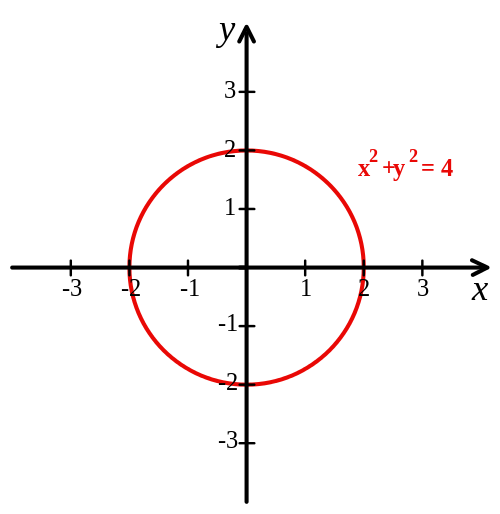# Cartesian Coordinate System

## What is a Cartesian Coordinate System?

A Cartesian Coordinate System is a system used to display a specified point defined in a plane by a set of numerical coordinates. The coordinates represent the point's distance from two, fixed perpendicular lines, known as axes (plural of axis). Both axes measure points in the same unit of length and extend across the real n-space. The point at which the two perpendicular axes intersect is known as the origin, defined with the ordered pair (0, 0). The vertical axis is defined as the y-axis, and the horizontal axis is defined as the x-axis.Source

## How does a Cartesian Coordinate System Work?

Cartesian Coordinate System was initially invented by Rene Descartes in the 17th century. The system revolutionized mathematics as it provided a link between Greek Euclidean geometry and algebra. For example, a geometric shape like a circle can be defined on a Cartesian system with a Cartesian equation. A circle with a radius of two that is positioned over the origin of a Cartesian system would have points whose coordinates X and Y satisfy the equation x2 + y2 = 4. In short, geometric shapes can be presented atop a Cartesian System as long as they consist of points that can be defined by the two axes.Source

There are, however, several dimensions of Cartesian Coordinate Systems. A one dimensional system can be described simply as a number line, in which every real number is defined by a unique location on the line in an ordered continuum. The two dimensional Cartesian Coordinate System is the commonly understood iteration, in which there are defined X and Y axes, and points upon the system are defined by an ordered pair of numbers. In cases in which a point is defined in three dimensional space, a Cartesian Coordinate System is defined by an X-axis, a Y-axis, and also a Z-axis. Each of the axes still intersect upon the common origin, and are also still perpendicular to each other. In three dimensional space, however, a point is defined by its distance not only to the X and Y axes, but also its distance from the other two axis, as defined by the Z-axis. The standard notation for three dimensional points is (x, y, z).

A standard two dimensional Cartesian Coordinate System divides the plane into four infinite regions known as quadrants. The quadrants, often denotes by roman numerals from 1 to 4, are defined in a counter-clockwise manner, where the first quadrant is defined as the upper, righthand space. Accordingly, a three dimensional system divides the plane into not four, but eight regions, known as octants.

### Applications of Cartesian Coordinate Systems

An easily understood example of the use of Cartesian coordinates is a standard map of the Earth. If latitude and longitude are defined along the X and Y axes, and the radius of the earth along the Z-axis, one can define any point on Earth with three dimensional geocentric coordinates. This method for geographic positioning is the basis for modern GPS navigation. It is important to note that the efficacy of Cartesian Coordinate Systems depends, almost entirely, on a universally accepted definition of the units defined along each Cartesian axis. For example, GPS would not work at all if each country defined the global origin differently. Currently, it is defined by the gravitational center of the Earth.